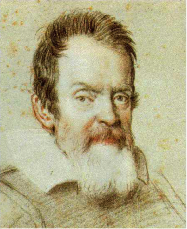Forces and Motion

Equation 1.1

velocity = distance      time

v =  d—t

Equation 1.2

time = distance      velocity

t = d —v

Speed and Velocity

Notebook

Equations

s = dt

(speed =

distance/time)

v = d—t

(velocity is vector so

includes direction; velocity = distance/time)

Can solve Eqn. 1 for others terms:

t = dv      and      d = vt

Memory AidCover the unknown and what is left is the right equation!Notes

• ALL SPEEDS ARE AVERAGE SPEEDS

• Speed = d/t

• Speed is a scalar quantity.

• Velocity is a vector quantity; v = d/t.

• A trip to Mars would take around 8 months.

• Stars are trillions of kilometers away

• Who is the guy below?

Galileo who first studied motion in 1600s.

• 8,760 hours in a year